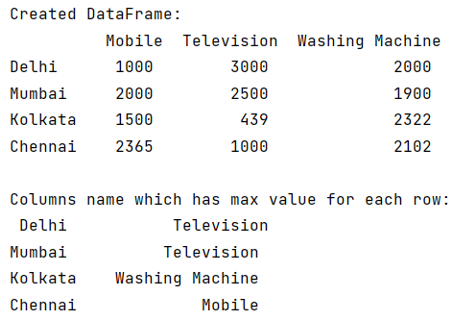# Find the column name which has the maximum value for each row

Given a Pandas DataFrame, we have to find the column name which has the maximum value for each row.
Submitted by Pranit Sharma, on June 13, 2022

Columns are the different fields that contain their particular values when we create a DataFrame. We can perform certain operations on both rows & column values.

Whereas rows in pandas are the different cell (column) values that are aligned horizontally and also provide uniformity. Each row can have the same or different value. Rows are generally marked with the index number but in pandas, we can also assign index names according to the needs. In pandas, we can create, read, update and delete a column or row value.

To access the column name which has the maximum value for each row, we will use dataframe.idmax() method.

To work with pandas, we need to import pandas package first, below is the syntax:

```import pandas as pd
```

Let us understand with the help of an example,

## Python code to find the column name which has the maximum value for each row

```# Importing pandas package
import pandas as pd

# Creating a dictionary
d = {
'Mobile':[1000,2000,1500,2365],
'Television':[3000,2500,439,1000],
'Washing Machine':[2000,1900,2322,2102]
}

# Creating a DataFrame
df = pd.DataFrame(d,index=['Delhi','Mumbai','Kolkata','Chennai'])

# Display DataFrame
print("Created DataFrame:\n",df,"\n")

# Returning column name which has
# max value for each row
result = df.idxmax(axis=1)

# Display result
print("Columns name which has max value for each row:\n",result)
```

Output:What's New (MCQs)

Top Interview Coding Problems/Challenges!

IncludeHelp's Blogs

Languages: » C » C++ » C++ STL » Java » Data Structure » C#.Net » Android » Kotlin » SQL
Web Technologies: » PHP » Python » JavaScript » CSS » Ajax » Node.js » Web programming/HTML
Solved programs: » C » C++ » DS » Java » C#
Aptitude que. & ans.: » C » C++ » Java » DBMS
Interview que. & ans.: » C » Embedded C » Java » SEO » HR
CS Subjects: » CS Basics » O.S. » Networks » DBMS » Embedded Systems » Cloud Computing
» Machine learning » CS Organizations » Linux » DOS
More: » Articles » Puzzles » News/Updates